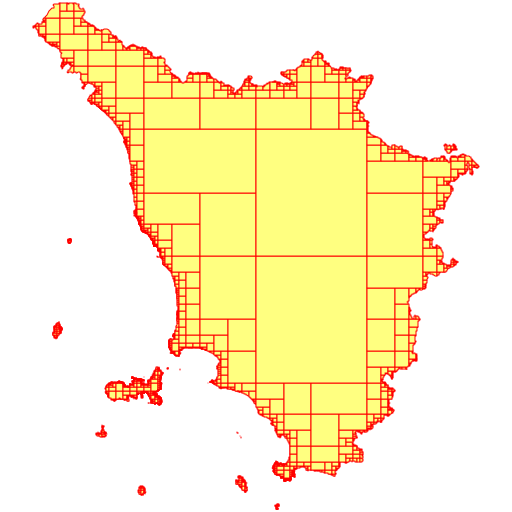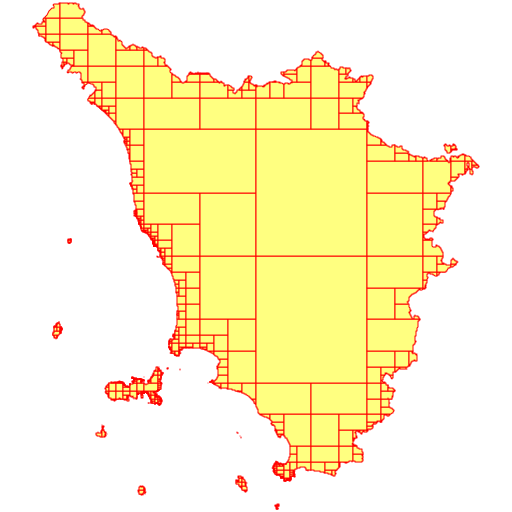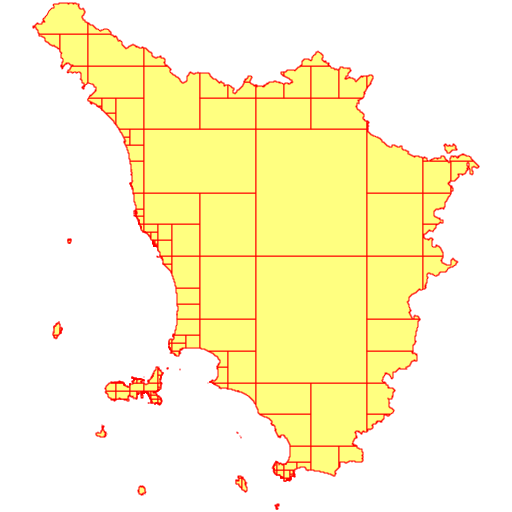Not logged in

# ST_Subdivide(): a quick intro

 Back to 5.0.0-doc main page

Starting with version 5.0.0 SpatiaLite supports ST_Subdivide(), an advanced SQL function already available on PostGIS.
The implementation is very similar in both Spatial DBMSes because in PostGIS the function is created with the internal lwgeom library, and in SpatiaLite with librttopo, which is a universal porting of lwgeom without PostGIS.

A short rationale: processing huge geometries having an impressive number of Vertices (many thousands or even more) is an extremely slow process.
Subdividing them into many smaller parts (while preserving full topological consistency) usually results in a satisfying processing speed. This is exactly the intended scope of ST_Subdivide():
• this function will receive an input geometry (which may very well be extremely large).
• each Linestring or Polygon found within the input geometry will be processed:
• all Linestrings or Polygons using a number of Vertices lesser or equal than the given threshold will be returned as they are.
• everything else will be recursively split, until a collection of elementary parts exists, using no more than the required number of vertices.
• when compleated, the function will return one geometry as a collection containing all of the elementary parts (a MultiLinestring or a MultiPolygon depending on the nature of the input geometry).

## Basic Samples

The following sampless are based on the, freely available, am_reg_multipart Shapefile and can be download from here here (search for Ambiti Amministrativi - Administrative Boundaries).

This Shapefile supports a very accurate and precise map representation, and the main exterior ring has more than 100,000+ Vertices.
It's a very good example of a Geometry, that is so huge and will cause any Spatial operator, such as ST_Intersects, ST_Touches, ST_Covers etc., to become extremely slow.

SQL queryVisual Sample
```SELECT ST_Subdivide(geometry) FROM tuscany;
```
in this first call, with no optional argument specified, ST_Subdivide() will start splitting after use the standard threshold of 128 Vertices is exceeded.

As the side figure shows, the returned result is a collection of about 4,300+ elementary parts.```SELECT ST_Subdivide(geometry, 512) FROM tuscany;
```
in this second call the optional argument is explicitly set, and ST_Subdivide() is now assuming a threshold of 512 Vertices.

As the side figure shows, the returned result is a collection of about 1,800+ elementary parts.```SELECT ST_Subdivide(geometry, 2048) FROM tuscany;
```
in this third and final call the threshold is set to start after 2,048 Vertices.

As shown by the figure, the returned result is now a collection containing only 1,200+ elementary parts.

### Short conclusion

ST_Subdivide() is very capable of subdividing a huge, nasty, Geometry into a collection of smaller parts, that can be handled in a more reasonable manner.
And it's ay flexible and easy to customize; you just have to set an appropriate Vertices threshold.However, a big disadvantage should be always be considered when using the multi-part collections returned by ST_Subdivide().
At least in the case of MultiPolygons, any collection returned by ST_Subdivide() is inherently invalid, as the following SQL query demonstrates:
```SELECT ST_IsValid(ST_Subdivide(geometry)) FROM tuscany;
---------
0
```
It's not at all difficult to understand why this is so.
Accordingly to standard OGC/SFS rules: two single-part Polygons, belonging to the same MultiPolygon, are always forbidden to share a common border.
More precisely: they can only touch on specific point(s), but they can never share a common boundary.

### ¡¡¡ Warning !!!

Never, ever, pass such a Geometry to any Spatial operator such as ST_Intersects, ST_Touches, ST_Covers etc..

This will certainly drive the GEOS library nutty.
Incorrect results may easily follow.
And in the worst case some unexpected crash could eventually occur.

### You have been warned !!!

Now we'll go on to explore the real effectiveness of ST_Subdivide() for one of the most common Spatial Problems: identifying all intersections between a dataset of the POINT type and a huge Polygon/MultiPolygon.

During this second test we'll use the following datasets:
• using, once again, the same administrative boundary of Tuscany we've already used before.
• for the POINT dataset we'll use the civici Shapefile (House Numbers)), also freely available, and can be download here here (search for Grafo Stradale - Road Network).
This too is a huge dataset containing about 1.5 million points.

### A first simple attempt

```SELECT Count(*)
FROM civici AS c
LEFT JOIN tuscany AS t ON (ST_Intersects(c.geometry, t.geometry) = 1);

SELECT Count(*)
FROM civici AS c
LEFT JOIN tuscany AS t ON (ST_Intersects(c.geometry, t.geometry) = 1
AND c.rowid IN (
SELECT rowid FROM SpatialIndex
WHERE f_table_name = 'civici' AND search_frame = t.geometry));
```
The only difference between the two SQL queries is in that the second one explicitly calls the Spatial Index.

Actual findings:
• I was forced to prematurely abort the first test when I discovered that, during the first half hour, it had made little progress.
My estimated conclusion was that between 4 and 6 hours would be required for completion.
Definitely this isn't a practical solution for our problem.
• the second test was, if possible, even slower than the first one.
And for very good reasons, that are easy to explain; all Points to be checked fall inside the BBOX of Tuscany.
So the Spatial Index isn't of any possible help.
And to make matters worst, querying the Spatial Index imposes a further overhead, thus requiring a far longer time to achieve absolutely nothing.
• Short conclusion: computing millions of intersections between a Point and a Polygon, containing hundreds of thousands of Vertices, is a complex computational problem.
Requiring such a very long time, makes such a solution absolutely unpractical, so a new approch to solve this problem is needed.

### A second attempt based on ST_Subdivide() and VirtualElementary

```CREATE TABLE xx (id INTEGER PRIMARY KEY);
SELECT AddGeometryColumn('xx', 'geom', 3003, 'MULTIPOLYGON', 'XY');
INSERT INTO xx VALUES (1, NULL);
```
We'll start first by creating a convenience Table, which will store the result of ST_Subdivide().
This is a very simple Table:
• it has just two columns, a Primary Key and a MultiPolygon Geometry.
• and will store only a single row identified by id=1
```UPDATE xx SET geom = (SELECT ST_Subdivide(geometry, 2048) FROM tuscany) WHERE id = 1;
SELECT Count(*)
FROM ElementaryGeometries AS t
JOIN civici AS c ON (ST_Intersects(c.geometry, t.geometry) = 1
AND c.rowid IN (
SELECT rowid FROM SpatialIndex
WHERE f_table_name = 'civici' AND search_frame = t.geometry))
WHERE f_table_name = 'xx' AND origin_rowid = 1;
----------------
1480991 (in 38.094 seconds)

UPDATE xx SET geom = (SELECT ST_Subdivide(geometry, 512) FROM tuscany) WHERE id = 1;
SELECT Count(*)
FROM ElementaryGeometries AS t
JOIN civici AS c ON (ST_Intersects(c.geometry, t.geometry) = 1
AND c.rowid IN (
SELECT rowid FROM SpatialIndex
WHERE f_table_name = 'civici' AND search_frame = t.geometry))
WHERE f_table_name = 'xx' AND origin_rowid = 1;
----------------
1480991 (in 12.617 seconds)

UPDATE xx SET geom = (SELECT ST_Subdivide(geometry) FROM tuscany) WHERE id = 1;
SELECT Count(*)
FROM ElementaryGeometries AS t
JOIN civici AS c ON (ST_Intersects(c.geometry, t.geometry) = 1
AND c.rowid IN (
SELECT rowid FROM SpatialIndex
WHERE f_table_name = 'civici' AND search_frame = t.geometry))
WHERE f_table_name = 'xx' AND origin_rowid = 1;
----------------
1480991 (in 9.534 seconds)
```
Quick evaluation:
• ST_Subdivide definitely is the tool of the trade we were searching for.
It effectively allows to resolve a computationally complex problem in a surprisingly quick time.
From many long hours, to just a handful of seconds, is a singularly astonishing improvement.
• subdividing the initial huge Polygon into many simpler/smaller parts has several beneficial effects:
• the time required to compute a point-to-polygon intersection quickly increases with the number of Vertices.
Smaller polygons are obviously swifter to be evaluated than bigger ones.
• smaller polygons can be an efficient Spatial Index filter.
• using VirtualElementary, in order to process all sub-areas one by one, surely imposes further complexity to our SQL queries.
But in the end, this method proves itself to be a very efficient mechanism to resolve this complex problem.
• Note: SpatiaLite is not PostGIS, and SQLite3 is not PostgreSQL; their respective internal architectures significantly differ in many ways.
More specifically, SQLite is completely unable to support arrays or multi-row results, so using VirtualElementary is an absolutely necessary workaround.

### A final experimental attempt based on a pure SQL approach

Another alternative mechanism, based on pure SQL and not requiring the use of ElementaryGeometries or of a convenience Table is:
```WITH RECURSIVE magic(n, geom) AS (
VALUES(1, (SELECT ST_Subdivide(geometry, 2048) FROM tuscany))
UNION ALL
SELECT n + 1, geom
FROM geom_ind
WHERE ST_GeometryN(geom, n) IS NOT NULL
)
SELECT n, ST_GeometryN(geom, n) FROM magic;
```
• we simply have to write a Recursive SQL Query in order to get a multi-row resultset, with a distinct row for each elementary part.
• it effectively works, but seems to be slower than ElementaryGeometries.
• and even more relevant, I was unable to write a complete Query determining all intersections between Points and Polygons in a reasonable time.
I suspect that the Query Optimizer of SQLite simply ignores the Spatial Index, thus ruining any advantage that this approach would bring.
It may well be, that someone else could succeed where I failed; any further third-party investigation on this specific area would be surely welcome.

 Back to 5.0.0-doc main page